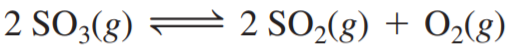×
Get Full Access to Chemistry: A Molecular Approach - 3 Edition - Chapter 14 - Problem 99e
Get Full Access to Chemistry: A Molecular Approach - 3 Edition - Chapter 14 - Problem 99e

×

# A sample of SO3 is introduced into an evacuated sealedISBN: 9780321809247 1

## Solution for problem 99E Chapter 14

Chemistry: A Molecular Approach | 3rd Edition

• Textbook Solutions
• 2901 Step-by-step solutions solved by professors and subject experts
• Get 24/7 help from StudySoup virtual teaching assistantsChemistry: A Molecular Approach | 3rd Edition

4 5 1 352 Reviews
14
4
Problem 99E

A sample of SO3 is introduced into an evacuated sealed container and heated to 600 K. The following equilibrium is established:The total pressure in the system is 3.0 atm and the mole fraction of O2 is 0.12. Find Kp.

Step-by-Step Solution:

Solution 99EStep 1The given chemical reaction.The equilibrium expression for the given reaction.In terms to pressure.

Step 2 of 5

Step 3 of 5

##### ISBN: 9780321809247

This full solution covers the following key subjects: atm, container, Equilibrium, established, evacuated. This expansive textbook survival guide covers 82 chapters, and 9454 solutions. This textbook survival guide was created for the textbook: Chemistry: A Molecular Approach, edition: 3. The full step-by-step solution to problem: 99E from chapter: 14 was answered by , our top Chemistry solution expert on 02/22/17, 04:35PM. Since the solution to 99E from 14 chapter was answered, more than 396 students have viewed the full step-by-step answer. Chemistry: A Molecular Approach was written by and is associated to the ISBN: 9780321809247. The answer to “?A sample of SO3 is introduced into an evacuated sealed container and heated to 600 K. The following equilibrium is established: The total pressure in the system is 3.0 atm and the mole fraction of O2 is 0.12. Find Kp.” is broken down into a number of easy to follow steps, and 40 words.

Unlock Textbook Solution